0
4154

# Syllogism Questions For IBPS Clerk PDF:

Download Syllogism Questions For IBPS Clerk PDF. Practice Important IBPS Clerk Questions and Answers for Banking Exams based on asked questions in previous papers.

Take Free IBPS Clerk Mock Test

Go to Free Banking Study Material (15,000 Solved Questions)

Instructions:

In each of the questions below are given four statements followed by four conclusions numbered I, II, III & IV. You have to take the given statements to be true even if they seem to be at given conclusions logically follows from the given statements disregarding commonly known facts.

Question 1: Statements:
All cups are bottles.
Some bottles are jugs.
No jug is plate.
Some plates are tables. Conclusions:
I. Some tables are bottles.
II. Some plates are cups.
III. No table is bottle.
IV. Some jugs are cups.

a) Only I follows

b) Only II follows

c) Only III follows

d) Only IV follows

e) Only either I or III follows

Question 2: Statements: Some chairs are handles. All handles are pots. All pots are mats. Some mats are buses. Conclusions: I. Some buses are handles. II. Some mats are chairs. III. No bus is handle. IV. Some mats are handles.

a) Only I, II and IV follow

b) Only II, III and IV follow

c) Only either I or III and II follow

d) Only either I or III and IV follow

e) Only either I or III and II and IV follow

Question 3: Statements: All birds are horses. All horses are tigers. Some tigers are lions. Some lions are monkeys. Conclusions: I. Some tigers are horses. II. Some monkeys are birds. III. Some tigers are birds. IV. Some monkeys are horses.

a) Only I and III follow

b) Only I, II and III follow

c) Only II, III and IV follow

d) All I, II, III and IV follow

e) None of these

Question 4: Statements: Some benches are walls. All walls are houses. Some houses are jungles. All jungles are roads. Conclusions: I. Some roads are benches. II. Some jungles are walls. III. Some houses are benches. IV. Some roads are houses.

a) Only land II follow

b) Only I and III follow

c) Only III and IV follow

d) Only II, III and IV follow

e) None of these

Question 5: Statements: Some sticks are lamps. Some flowers are lamps. Some lamps are dresses. All dresses are shirts. Conclusions: I. Some shirts are sticks. II. Some shirts are flowers. III. Some flowers are sticks. IV. Some dresses are sticks.

a) None follows

b) Only I follows

c) Only II follows

d) Only III follows

e) Only IV follows

Instructions:

In each of the questions below, two/three statements are given followed by conclusions/ group of conclusions numbered I and II. You have to assume all the statements to be true even if they seem to be at variance with the commonly known facts and then decide which of the given two conclusions logically follows from the information given in the statements.

Question 6: Statements:
Some squares are circles.
No circle is a triangle.
No line is a square. Conclusions:
I. All squares can never be triangles.
II. Some lines are circles.

a) Only conclusion I follows.

b) Only conclusion II follows.

c) Either conclusion I or conclusion II follows.

d) Neither conclusion I nor conclusion II follows.

e) Both conclusion I and II follow.

Question 7: Statements:
Some squares are circles.
No circle is a triangle.
No line is a square. Conclusions:
I. No triangle is a square.
II. No line is a circle

a) Only conclusion I follows.

b) Only conclusion II follows.

c) Either conclusion I or conclusion II follows.

d) Neither conclusion I nor conclusion II follows.

e) Both conclusion I and II follow.

Question 8: Statements:
All songs are poems.
All poems are rhymes.
No rhyme is paragraph. Conclusions:
I. No Song is a paragraph.
II. No poem is a paragraph.

a) Only conclusion I follows.

b) Only conclusion II follows.

c) Either conclusion I or conclusion II follows.

d) Neither conclusion I nor conclusion II follows.

e) Both conclusion I and II follow.

Question 9: Statements:
All songs are poems.
All poems are rhymes.
No rhyme is paragraph. Conclusions:
I. All rhymes are poems.
II. All Songs are rhymes.

a) Only conclusion I follows.

b) Only conclusion II follows.

c) Either conclusion I or conclusion II follows.

d) Neither conclusion I nor conclusion II follows.

e) Both conclusion I and II follow.

Question 10: Statements:
Some dews are drops.
All drops are stones. Conclusions:
I. At least some dews are stones.
II. At least some stones are drops.

a) Only conclusion I follows.

b) Only conclusion II follows.

c) Either conclusion I or conclusion II follows.

d) Neither conclusion I nor conclusion II follows.

e) Both conclusion I and II follow.

Instructions:

In each question below are given two/three statements followed by two conclusions numbered I and II. You have to take the given statements to be true even if they seem to be at variance with commonly known facts and then decide which of the given conclusions logically follows from the given statements disregarding commonly known facts. Give answer
A: if only conclusion I follows.
B: if only conclusion II follows.
C: if either conclusion I or conclusion II follows.
D: if neither conclusion I nor conclusion II follows.
E: if both conclusion I and conclusion II follow.

Question 11: Statements:
All gliders are parachutes.
No parachute is an airplane.
All airplanes are helicopters.
Conclusions:
I. No helicopter is a glider.
II. All parachutes being helicopters is a possibility.

a) if only conclusion I follows.

b) if only conclusion II follows.

c) if either conclusion I or conclusion II follows.

d) if neither conclusion I nor conclusion II follows.

e) if both conclusion I and conclusion II follow.

Question 12: Statements:
All gliders are parachutes.
No parachute is an airplane.
All airplanes are helicopters.
Conclusions:
I. No glider is an airplane.
II. All gliders being helicopters is a possibility.

a) if only conclusion I follows.

b) if only conclusion II follows.

c) if either conclusion I or conclusion II follows.

d) if neither conclusion I nor conclusion II follows.

e) if both conclusion I and conclusion II follow.

Question 13: Statements:
Some mails are chats.
Conclusions:
I All mails being updates is a possibility.
II. No update is a mail.

a) if only conclusion I follows.

b) if only conclusion II follows.

c) if either conclusion I or conclusion II follows.

d) if neither conclusion I nor conclusion II follows.

e) if both conclusion I and conclusion II follow.

Question 14: Statements:
No stone is metal.
Some metals are papers.
All papers are glass.
Conclusions:
I. No glass is metal.
II. At least some glasses are metals.

a) if only conclusion I follows.

b) if only conclusion II follows.

c) if either conclusion I or conclusion II follows.

d) if neither conclusion I nor conclusion II follows.

e) if both conclusion I and conclusion II follow.

Question 15: Statements:
No stone is metal.
Some metals are papers.
All papers are glass.
Conclusions:
I. All stones being glass is a possibility.
II. No stone is paper.

a) if only conclusion I follows.

b) if only conclusion II follows.

c) if either conclusion I or conclusion II follows.

d) if neither conclusion I nor conclusion II follows.

e) if both conclusion I and conclusion II follow.

Question 16: Statements:
Some exams are tests.
No exam is a question.
Conclusions:
I. No question is a test.
II. Some tests are definitely not exams.

a) If only conclusion I follows

b) If only conclusion II follows

c) If either conclusion I or conclusion II follows

d) If neither conclusion I nor conclusion II follows

e) If both conclusion I and conclusion II follow

Question 17: Statements:
All forces are energies.
All energies are powers.
No power is heat.
Conclusions:
I. Some forces are definitely not powers.
II. No heat is force.

a) If only conclusion I follows

b) If only conclusion II follows

c) If either conclusion I or conclusion II follows

d) If neither conclusion I nor conclusion II follows

e) If both conclusion I and conclusion II follow

Question 18: Statements:
All forces are energies.
All energies are powers.
No power is heat.
Conclusions:
I. No energy is heat.
II. Some forces being heat is a possibility.

a) If only conclusion I follows

b) If only conclusion II follows

c) If either conclusion I or conclusion II follows

d) If neither conclusion I nor conclusion II follows

e) If both conclusion I and conclusion II follow

Question 19: Statements:
No note is a coin.
Some coins are metals.
All plastics are notes.
Conclusions:
I. No coin is plastic.
II. All plastics being metals is a possibility.

a) If only conclusion I follows

b) If only conclusion II follows

c) If either conclusion I or conclusion II follows

d) If neither conclusion I nor conclusion II follows

e) If both conclusion I and conclusion II follow

Question 20: Statements:
No note is a coin.
Some coins are metals.
All plastics are notes.
Conclusions:
I. No metal is plastic.
II. All notes are plastics.

a) If only conclusion I follows

b) If only conclusion II follows

c) If either conclusion I or conclusion II follows

d) If neither conclusion I nor conclusion II follows

e) If both conclusion I and conclusion II follow

The conclusions talk about a relation between tables and bottles. Let’s bring a relation between these two using the given statements. Some bottles are jugs and no jug is a plate => some bottles can be plates or no bottle is a plate Some plates are tables => some bottles can be plates or no bottle is a plate. — (1) All cups are bottles and some bottles are jugs => Nothing about cups and jugs can be derived from this. Since we cannot derive a conclusion between cups and jugs, it is not possible to derive a conclusion between cups and plates as well. Hence option E, which says that some bottles can be plates or no bottle is a plate is the answer.

The following are the two possibilities: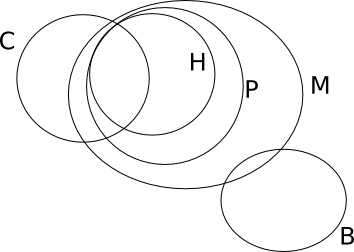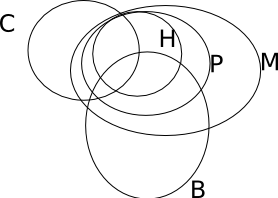So, either I or III and II and IV follow.

Birds is a subset of Horses, which is a subset of Tigers. So, conclusions I and III follow. But, conclusions II and IV need not follow.

Based on the following Venn diagram, only conclusions 3 and 4 are implied.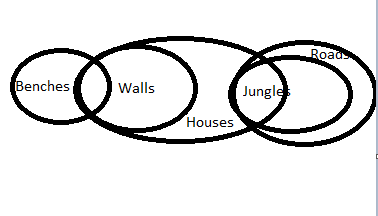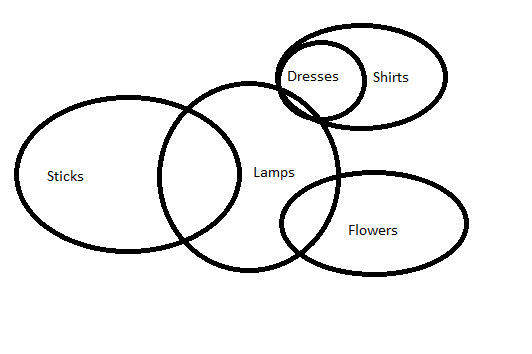Based on the Venn diagram, none of the conclusions are implied by the statements.

Some squares are circles and no circle is a triangle => Some squares are not triangles => Conclusion 1 follows No line is a square => No relation can be brought between line and circle => Conclusion 2 does not follow.

Some squares are circles and no circle is a triangle => Some squares are not triangles => Some squares being triangles is a possibility => Conclusion 1 does not follow No line is a square and some square are circle => Some lines being circles is a possibility => Conclusion 2 does not follow

All songs are poems and all poems are rhymes => All songs are rhymes No rhyme is a paragraph => No song is a paragraph => Conclusion 1 follows All poems are rhymes and no rhyme is a paragraph => No poem is a paragraph => Conclusion 2 follows

All poems are rhymes does not imply that all rhymes are poems. The correct conclusion is Some Rhymes are poems. Conclusion 1 does not follow. All songs are poems and all poems are rhymes => All songs are rhymes => Conclusion 2 follows

All drops are stones => Some stones are drops. So, conclusion II follows. Some dews are drops => Some drops are dews. But all drops are stones. => Some dews are stones. So, conclusion I follows. Option e) is the correct answer.

No parachute is an airplane and all airplanes are helicopters doesn’t give an indication about the relation between parachutes and helicopters. So, all parachutes are helicopters is not contradicted in the statements.

From the given statements, no glider is an airplane is implied but no relation is established between gliders and helicopters. So, all gliders being helicopters is a possibility.

Some mails are chats. There is a possibility that all mails are chats. All updates are chats. So, if mails is a subset of updates, which in turn is a subset of chats, the conditions still hold true. So, conclusion I follows. Conclusion II is not necessarily true as there might be some intersection between mails and updates.

Some metals are paper and all paper are glass => Some metals are glass or some glass are metal. So, conclusion II follows. No glass is metal need not be true. So, the correct answer is option b).

Consider the following diagram: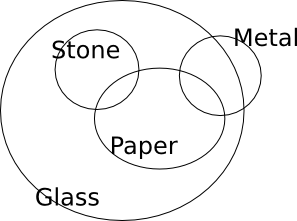From this we can see that all stones being glass is a possibility and no stone is paper need not be true. So, option a) is the correct answer.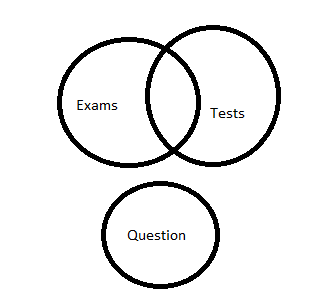Some exams are tests and no exam is a question. Nothing can be built between tests and questions from this information. Some exams are tests doesn’t mean some tests are not exams because this could be a case where all tests are exams. Hence neither of the statements is true.

All forces are energies and all energies are powers => all forces are powers. => conclusion 1 is wrong No power is a heat and all forces are powers => No heat is force. Only conclusion 2 follows.